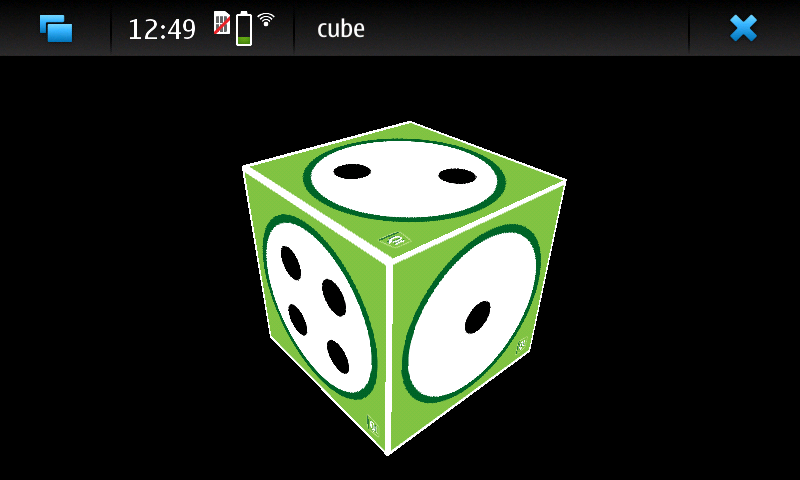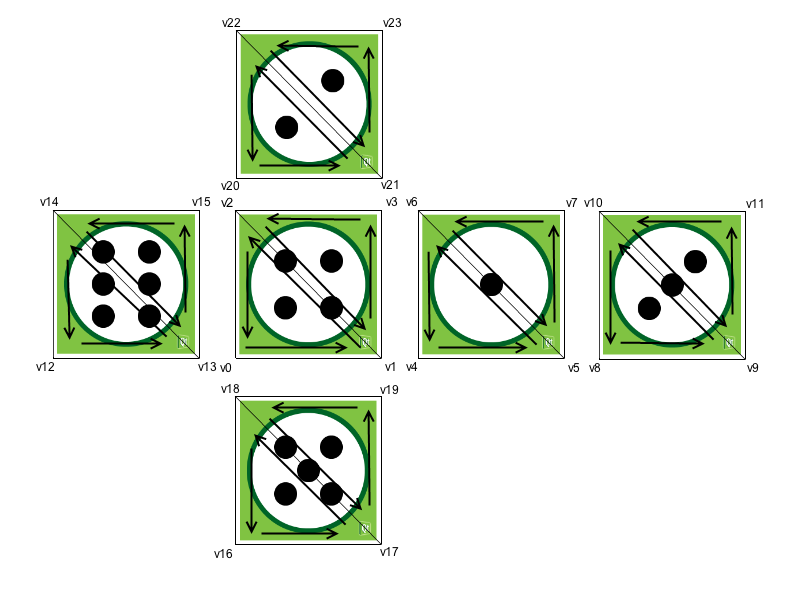# Cube OpenGL ES 2.0 example

Shows how to manually rotate a textured 3D cube with user input.

The Cube OpenGL ES 2.0 example shows how to manually rotate a textured 3D cube with user input, using OpenGL ES 2.0 with Qt. It shows how to handle polygon geometries efficiently and how to write a simple vertex and fragment shader for a programmable graphics pipeline. In addition it shows how to use quaternions for representing 3D object orientation.

This example has been written for OpenGL ES 2.0 but it works also on desktop OpenGL because this example is simple enough and for the most parts desktop OpenGL API is same. It compiles also without OpenGL support but then it just shows a label stating that OpenGL support is required.The example consist of two classes:

• `MainWidget` extends QOpenGLWidget and contains OpenGL ES 2.0 initialization and drawing and mouse and timer event handling
• `GeometryEngine` handles polygon geometries. Transfers polygon geometry to vertex buffer objects and draws geometries from vertex buffer objects.

We'll start by initializing OpenGL ES 2.0 in `MainWidget`.

#### Initializing OpenGL ES 2.0

Since OpenGL ES 2.0 doesn't support fixed graphics pipeline anymore it has to be implemented by ourselves. This makes graphics pipeline very flexible but in the same time it becomes more difficult because user has to implement graphics pipeline to get even the simplest example running. It also makes graphics pipeline more efficient because user can decide what kind of pipeline is needed for the application.

First we have to implement vertex shader. It gets vertex data and model-view-projection matrix (MVP) as parameters. It transforms vertex position using MVP matrix to screen space and passes texture coordinate to fragment shader. Texture coordinate will be automatically interpolated on polygon faces.

```void main()
{
// Calculate vertex position in screen space
gl_Position = mvp_matrix * a_position;

// Pass texture coordinate to fragment shader
// Value will be automatically interpolated to fragments inside polygon faces
v_texcoord = a_texcoord;
}```

After that we need to implement second part of the graphics pipeline - fragment shader. For this exercise we need to implement fragment shader that handles texturing. It gets interpolated texture coordinate as a parameter and looks up fragment color from the given texture.

```void main()
{
// Set fragment color from texture
gl_FragColor = texture2D(texture, v_texcoord);
}```

Using QOpenGLShaderProgram we can compile, link and bind shader code to graphics pipeline. This code uses Qt Resource files to access shader source code.

```void MainWidget::initShaders()
{
close();

close();

close();

// Bind shader pipeline for use
if (!program.bind())
close();
}```

The following code enables depth buffering and back face culling.

```    // Enable depth buffer
glEnable(GL_DEPTH_TEST);

// Enable back face culling
glEnable(GL_CULL_FACE);```

The `QOpenGLWidget` interface implements methods for loading textures from QImage to OpenGL texture memory. We still need to use OpenGL provided functions for specifying the OpenGL texture unit and configuring texture filtering options.

```void MainWidget::initTextures()
{
texture = new QOpenGLTexture(QImage(":/cube.png").mirrored());

// Set nearest filtering mode for texture minification
texture->setMinificationFilter(QOpenGLTexture::Nearest);

// Set bilinear filtering mode for texture magnification
texture->setMagnificationFilter(QOpenGLTexture::Linear);

// Wrap texture coordinates by repeating
// f.ex. texture coordinate (1.1, 1.2) is same as (0.1, 0.2)
texture->setWrapMode(QOpenGLTexture::Repeat);
}```

#### Cube Geometry

There are many ways to render polygons in OpenGL but the most efficient way is to use only triangle strip primitives and render vertices from graphics hardware memory. OpenGL has a mechanism to create buffer objects to this memory area and transfer vertex data to these buffers. In OpenGL terminology these are referred as Vertex Buffer Objects (VBO).This is how cube faces break down to triangles. Vertices are ordered this way to get vertex ordering correct using triangle strips. OpenGL determines triangle front and back face based on vertex ordering. By default OpenGL uses counter-clockwise order for front faces. This information is used by back face culling which improves rendering performance by not rendering back faces of the triangles. This way graphics pipeline can omit rendering sides of the triangle that aren't facing towards screen.

Creating vertex buffer objects and transferring data to them is quite simple using QOpenGLBuffer. MainWidget makes sure the GeometryEngine instance is created and destroyed with the OpenGL context current. This way we can use OpenGL resources in the constructor and perform proper cleanup in the destructor.

```GeometryEngine::GeometryEngine()
: indexBuf(QOpenGLBuffer::IndexBuffer)
{
initializeOpenGLFunctions();

// Generate 2 VBOs
arrayBuf.create();
indexBuf.create();

// Initializes cube geometry and transfers it to VBOs
initCubeGeometry();
}

GeometryEngine::~GeometryEngine()
{
arrayBuf.destroy();
indexBuf.destroy();
}
// Transfer vertex data to VBO 0
arrayBuf.bind();
arrayBuf.allocate(vertices, 24 * sizeof(VertexData));

// Transfer index data to VBO 1
indexBuf.bind();
indexBuf.allocate(indices, 34 * sizeof(GLushort));```

Drawing primitives from VBOs and telling programmable graphics pipeline how to locate vertex data requires few steps. First we need to bind VBOs to be used. After that we bind shader program attribute names and configure what kind of data it has in the bound VBO. Finally we'll draw triangle strip primitives using indices from the other VBO.

```void GeometryEngine::drawCubeGeometry(QOpenGLShaderProgram *program)
{
// Tell OpenGL which VBOs to use
arrayBuf.bind();
indexBuf.bind();

// Offset for position
quintptr offset = 0;

// Tell OpenGL programmable pipeline how to locate vertex position data
int vertexLocation = program->attributeLocation("a_position");
program->enableAttributeArray(vertexLocation);
program->setAttributeBuffer(vertexLocation, GL_FLOAT, offset, 3, sizeof(VertexData));

// Offset for texture coordinate
offset += sizeof(QVector3D);

// Tell OpenGL programmable pipeline how to locate vertex texture coordinate data
int texcoordLocation = program->attributeLocation("a_texcoord");
program->enableAttributeArray(texcoordLocation);
program->setAttributeBuffer(texcoordLocation, GL_FLOAT, offset, 2, sizeof(VertexData));

// Draw cube geometry using indices from VBO 1
glDrawElements(GL_TRIANGLE_STRIP, 34, GL_UNSIGNED_SHORT, nullptr);
}```

#### Perspective Projection

Using `QMatrix4x4` helper methods it's really easy to calculate perpective projection matrix. This matrix is used to project vertices to screen space.

```void MainWidget::resizeGL(int w, int h)
{
// Calculate aspect ratio
qreal aspect = qreal(w) / qreal(h ? h : 1);

// Set near plane to 3.0, far plane to 7.0, field of view 45 degrees
const qreal zNear = 3.0, zFar = 7.0, fov = 45.0;

// Reset projection
projection.setToIdentity();

// Set perspective projection
projection.perspective(fov, aspect, zNear, zFar);
}```

#### Orientation of the 3D Object

Quaternions are handy way to represent orientation of the 3D object. Quaternions involve quite complex mathematics but fortunately all the necessary mathematics behind quaternions is implemented in `QQuaternion`. That allows us to store cube orientation in quaternion and rotating cube around given axis is quite simple.

The following code calculates rotation axis and angular speed based on mouse events.

```void MainWidget::mousePressEvent(QMouseEvent *e)
{
// Save mouse press position
mousePressPosition = QVector2D(e->position());
}

void MainWidget::mouseReleaseEvent(QMouseEvent *e)
{
// Mouse release position - mouse press position
QVector2D diff = QVector2D(e->position()) - mousePressPosition;

// Rotation axis is perpendicular to the mouse position difference
// vector
QVector3D n = QVector3D(diff.y(), diff.x(), 0.0).normalized();

// Accelerate angular speed relative to the length of the mouse sweep
qreal acc = diff.length() / 100.0;

// Calculate new rotation axis as weighted sum
rotationAxis = (rotationAxis * angularSpeed + n * acc).normalized();

// Increase angular speed
angularSpeed += acc;
}```

`QBasicTimer` is used to animate scene and update cube orientation. Rotations can be concatenated simply by multiplying quaternions.

```void MainWidget::timerEvent(QTimerEvent *)
{
// Decrease angular speed (friction)
angularSpeed *= 0.99;

// Stop rotation when speed goes below threshold
if (angularSpeed < 0.01) {
angularSpeed = 0.0;
} else {
// Update rotation
rotation = QQuaternion::fromAxisAndAngle(rotationAxis, angularSpeed) * rotation;

// Request an update
update();
}
}```

Model-view matrix is calculated using the quaternion and by moving world by Z axis. This matrix is multiplied with the projection matrix to get MVP matrix for shader program.

```    // Calculate model view transformation
QMatrix4x4 matrix;
matrix.translate(0.0, 0.0, -5.0);
matrix.rotate(rotation);

// Set modelview-projection matrix
program.setUniformValue("mvp_matrix", projection * matrix);```

Example project @ code.qt.io